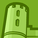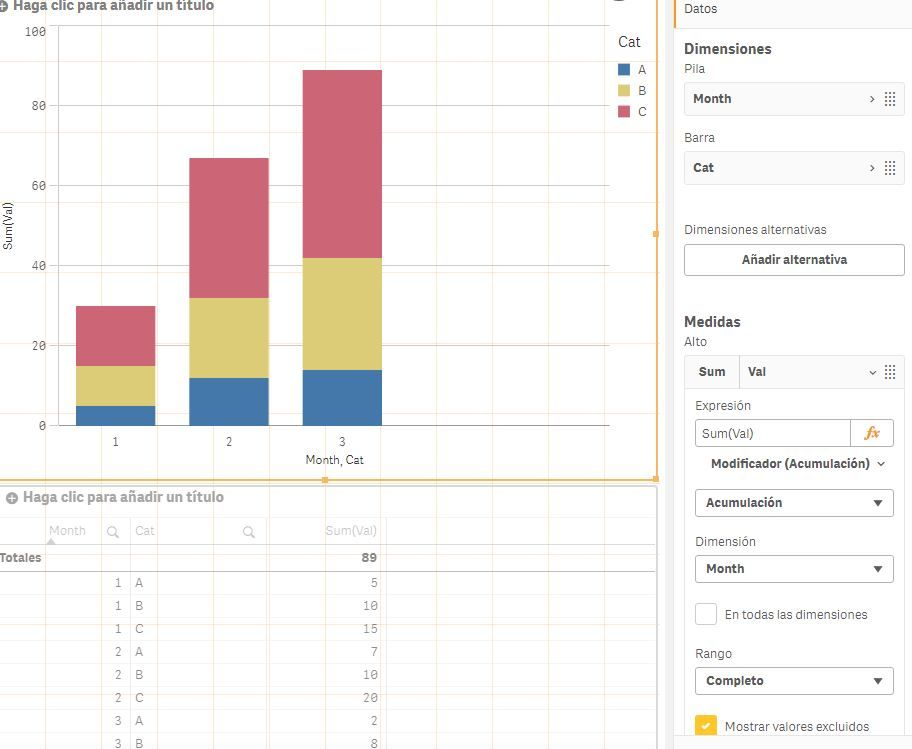# New to Qlik Sense

If you’re new to Qlik Sense, start with this Discussion Board and get up-to-speed quickly.

Announcements
cancel
Showing results for
Did you mean:Contributor III

## Stacked Bar Chart Two Dimensions Cumulated Values

Hi, I want to create a Stacked bar chart in Qlik Sense with cumulative values using two dimensions. Is this possible? We had one in QlikView and it looked like the attached image. I tried to use Rangesum(Above([Expression],0,rowno())) but it didn´t work.

2 Solutions

Accepted SolutionsMVP

Mine seems correct, and in your screenshot E has 400k, wich is correct with his Y axis, maybe you have an isse assigning values to each segment:Check my attached app to look for differences.MVP

That looks like an issue on the way accumulations are calculated, in this case I would suggest to create data so all combinations on Entry and month has data, this script assigns zero to the non-existant combinations:

``````Data:
NoConcatenate
LOAD Entry, Data, Dual(Month, NumMonth) as Month, Entry &'_'& Month as KeyLoaded;
LOAD *, Num(Month(Date#(Month, 'MMM'))) as NumMonth Resident Blad1 where Data<>0; // This 'where' is just to simulate the null value on Jul

// Generate all possible combinations between Entry and Month
Cartesian:
LOAD FieldValue('Entry', RecNo()) as Entry AutoGenerate FieldValueCount('Entry');
Join LOAD FieldValue('Month', RecNo()) as Month,
Num(Month(Date#(FieldValue('Month', RecNo()), 'MMM'))) as NumMonth
AutoGenerate FieldValueCount('Month');

Concatenate (Data)
LOAD Entry, 0 as Data, Dual(Month, NumMonth) as Month
Resident Cartesian where not exists('KeyLoaded', Entry &'_'& Month);

DROP table Cartesian;

10 RepliesMVP

Hi, february-2020 version has an option to apply modifiers, one of them is accumulation.

Using 2 stacked dimensions and a expression the expression it generates automatically is:

``Sum({1<[\$(=Replace(GetObjectField(0),']',']]'))]={">=\$(=Min([\$(=Replace(GetObjectField(0),']',']]'))]))<=\$(=Max([\$(=Replace(GetObjectField(0),']',']]'))]))"},[\$(=Replace(GetObjectField(1),']',']]'))]={"=Only({1}[\$(=Replace(GetObjectField(1),']',']]'))])>='\$(=MinString([\$(=Replace(GetObjectField(1),']',']]'))]))' and Only({1}[\$(=Replace(GetObjectField(1),']',']]'))])<='\$(=MaxString([\$(=Replace(GetObjectField(1),']',']]'))]))'"}>}Aggr(RangeSum(Above(If(Count([\$(=Replace(GetObjectField(0),']',']]'))]) * Count([\$(=Replace(GetObjectField(1),']',']]'))]) > 0,  (　Sum(Val)　)  + Sum({1<[\$(=Replace(GetObjectField(0),']',']]'))]={">=\$(=Min([\$(=Replace(GetObjectField(0),']',']]'))]))<=\$(=Max([\$(=Replace(GetObjectField(0),']',']]'))]))"},[\$(=Replace(GetObjectField(1),']',']]'))]={"=Only({1}[\$(=Replace(GetObjectField(1),']',']]'))])>='\$(=MinString([\$(=Replace(GetObjectField(1),']',']]'))]))' and Only({1}[\$(=Replace(GetObjectField(1),']',']]'))])<='\$(=MaxString([\$(=Replace(GetObjectField(1),']',']]'))]))'"}>}0), 0), 0, RowNo())), ([\$(=Replace(GetObjectField(1),']',']]'))], (Numeric, Ascending), (Text, Ascending)), ([\$(=Replace(GetObjectField(0),']',']]'))], (Numeric, Ascending), (Text, Ascending))))``Contributor III
Author

Ok, so I just have to wait for my IT department to update to the february 2020 release which is now planned on April 23. Sounds great! Thanks Rubenmarin, I´ll accept as solution as soon as it´s available and works for us.MVP

Meanwhile you can use the expression it creates automatically. I haven't tested in previous versions but it may work.Contributor III
Author

Finally we got the Feb release installed and I tried the new functionality. Didn´t get it to work properly though??? I attached some data and images. In these you can see that the size of the different stacks are wrong and the scale on the Y axis is wrong. When I hover over the stacks in the bars, the popup presents correct values.Partner

Hello,

Can u tell me what dimensions and measures u want on X axis and Y axis.

Regards,

Shivani SapkaleContributor III
Author

I want the months on the X axis, and the data (values) on the Y axis. Bars should be split as a stacked bar chart, with each stack displaying the accumulated sum of the Entry field.MVP

Mine seems correct, and in your screenshot E has 400k, wich is correct with his Y axis, maybe you have an isse assigning values to each segment:Check my attached app to look for differences.Contributor III
Author

Fantastic! Thank´s. This part was what was missing in my app

Data:
NoConcatenate
LOAD Entry, Data, Dual(Month, NumMonth) as Month;